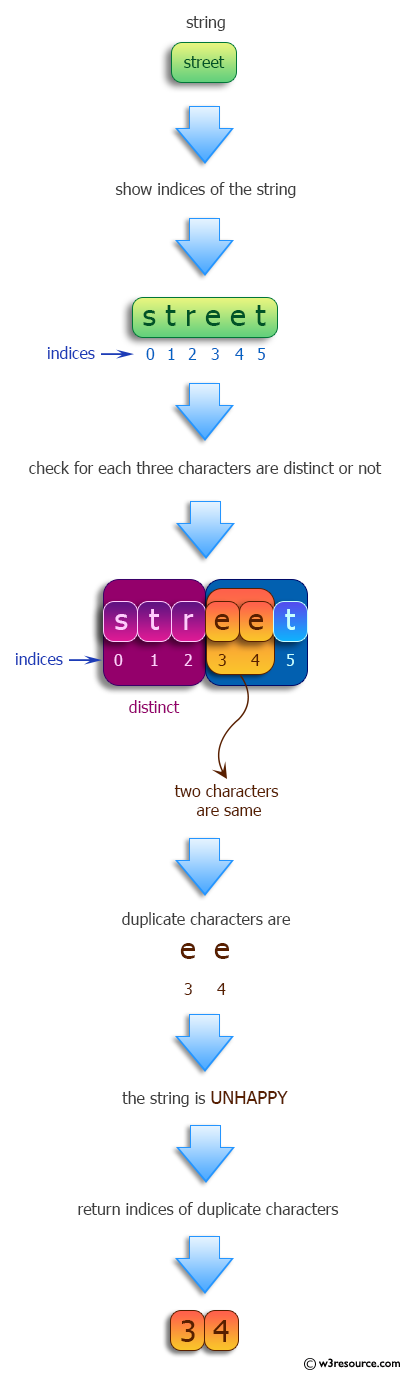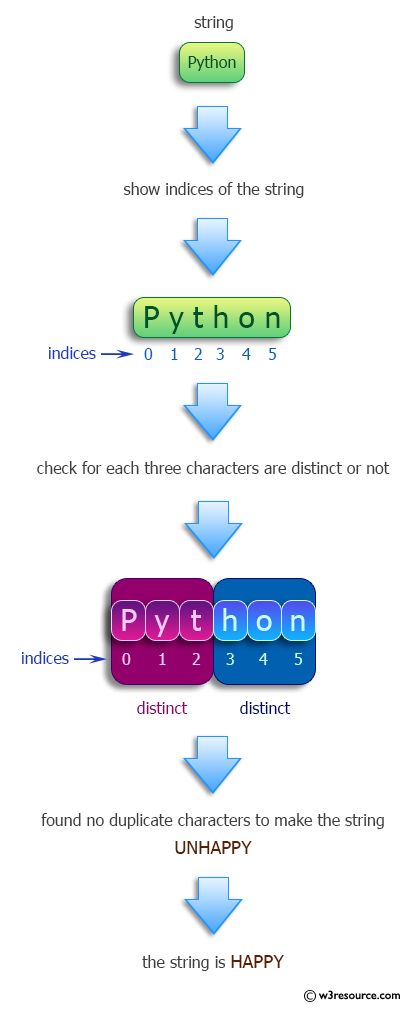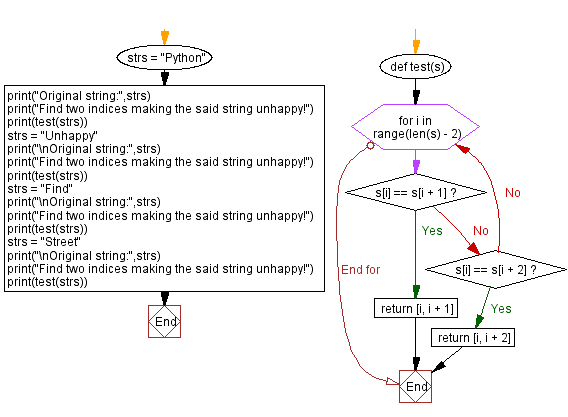﻿ Python: Find two indices making a given string unhappy - w3resource# Python: Find two indices making a given string unhappy

## Python Programming Puzzles: Exercise-83 with Solution

A string is happy if every three consecutive characters are distinct. Write a Python program to find two indices making a given string unhappy.

```Input:
Python
Output:
None

Input:
Unhappy
Output:
[4, 5]

Input:
Find
Output:
None

Input:
Street
Output:
[3, 4]
```

Pictorial Presentation:Sample Solution:

Python Code:

``````#License: https://bit.ly/3oLErEI

def test(s):
for i in range(len(s) - 2):
if s[i] == s[i + 1]:
return [i, i + 1]
if s[i] == s[i + 2]:
return [i, i + 2]

strs = "Python"
print("Original string:",strs)
print("Find two indices making the said string unhappy!")
print(test(strs))
strs = "Unhappy"
print("\nOriginal string:",strs)
print("Find two indices making the said string unhappy!")
print(test(strs))
strs = "Find"
print("\nOriginal string:",strs)
print("Find two indices making the said string unhappy!")
print(test(strs))
strs = "Street"
print("\nOriginal string:",strs)
print("Find two indices making the said string unhappy!")
print(test(strs))
``````

Sample Output:

```Original string: Python
Find two indices making the said string unhappy!
None

Original string: Unhappy
Find two indices making the said string unhappy!
[4, 5]

Original string: Find
Find two indices making the said string unhappy!
None

Original string: Street
Find two indices making the said string unhappy!
[3, 4]
```

Flowchart:## Visualize Python code execution:

The following tool visualize what the computer is doing step-by-step as it executes the said program:

Python Code Editor :

Have another way to solve this solution? Contribute your code (and comments) through Disqus.

What is the difficulty level of this exercise?

Test your Programming skills with w3resource's quiz.

﻿

## Python: Tips of the Day

Clamps num within the inclusive range specified by the boundary values x and y:

Example:

```def tips_clamp_num(num,x,y):
return max(min(num, max(x, y)), min(x, y))
print(tips_clamp_num(2, 4, 6))
print(tips_clamp_num(1, -1, -6))
```

Output:

```4
-1
```